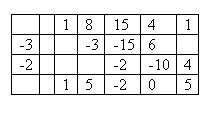## Monday, 2 February 2009

### Synthetic Divison by a Quadratic

I was sitting in a school improvement meeting paying less attention than I ought to, and started trying to figure out how to figure out the topic above. What is below is my way.. I tried to find something about this on the internet, but only found sites that deal with linear factors, and some of them said that what I have done was not possible (I hope they are wrong)... Then when I came home I found a post on the AP Calculus Discussion site that asked how to do this very thing, with a citation for an article in the Mathematics Teacher, (March, 1980 journal (hopefully the archives go back that far), there is an article called "Synthetic Division for Nonlinear Factors" ...thanks to Lisa Lewis) that describes the process. Unfortunatly I can't get that on-line.

So here is how I did it.. I made up a problem involving a fourth power polynomial, and a quadratic divisor(A clever student could break this divsor into two linear terms and do the same in two divisions... IF he is careful about remainders, but pretend I had been clever enough to use a irreducible quadratic for the divisor)

Divide x4 + 8x3+ 15x2 + 4x + 1 by x2 + 3x + 2, using synthetic division:

The dividend is written out in the usual order with the coefficients (including any missing zeros)... and to the left two columns with the negative of the B term (3 and the C term (2) as shown below... notice that I use the -B term above the -C term.. and as usual, the bottom row will be the quotient.The first step is still to bring down the leading coefficient.. from there there are two multiplications: the product of the -B term and the number in the bottom row go in the row with -B and the column with the last bottom number... The product of the -C term and the last bottom number will go in the column one to the right of the last bottom number. Note the locations of the -3 under the 8, and the -2 under the 15...Then we add up all the values in the second row to get a new bottom value.We continue this process, always keeping the product of the -C term entered one to the right of the last bottom number.Since we are dividing a fourth degree polynomial by a second degree polynomial, the answer will be of the second degree, and the last two cells on the bottom represent a linear remainder. In this case the quotient is x2 + 5x -2 and the remainder is 0x + 5... You can check the solution by multiplication.

Big Idea..... Gauss proved that all polynomials with real valued coefficients can be factored into a product of linear factors (like x-a) and irreducible quadratic factors (qudratics that don't have real roots, and hence can't be factored over the reals). So we can factor any real valued polynomial with nothing bigger than quadratic division....

But what if we have a seventh power polynomial and want to divide it by a cubic and we don't want to do it without breaking the cubic down... can it be done...?

OK, I don't know, but if I get a free moment in the next 24 hours, I think I will try extending this method to a third term and see if I get lucky...

Ok, so the remark about "breaking the cubic down" was not clear, so I will try to cover that in a day or two..... HEY, I have five classes to prep..give a guy a break...Anonymous said...

Mr. Ballew,
would you explain what you mean by breaking up the cubic... is that another way to do it?Anonymous said...

What do you do if one or both of the polynomials have a first number other than one.. like dividing by 2x^2 +x+1 or somthing.. what do I do with the 2?
Can those just not be done?Anonymous said...

Sarah,

I assume you can factor out a, and then divide the quotient by it.

Instead of dividing by 3x^2 - 5x + 6,
divide by x^2 - (5/3)x + 2, and then divide the quotient and remainder by 3.

In general I prefer long division to synthetic, but occasionally I'll use synthetic. In these cases though, isn't the familiarity with the algorithm from arithmetic a tremendous incentive to stick there?

Jonathan

Wakwak said...

thank you so much! this blog was really a great help...keep it up!

Unknown said...

Thanks pat, it was really helpful I'll do a little more practice on it to find out more about it hope I'll get some help from you , if you have done some work on cubic one please send me so that we could do it together and may be we succeed

Unknown said...

Dear pat, this is daksh who wrote this comment as "unknown". You can contact me here - dakshsinha7631@gmail.com

Unknown said...

What if the divisor was something like 3x^2+x+1 or x^2-6x+9

Unknown said...

how you can extend the use of synthetic division in dividing polynomial by a quadratic expression of x² + bx + c?

ErrorFinder said...

Wow! Amazing